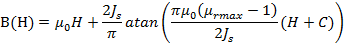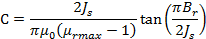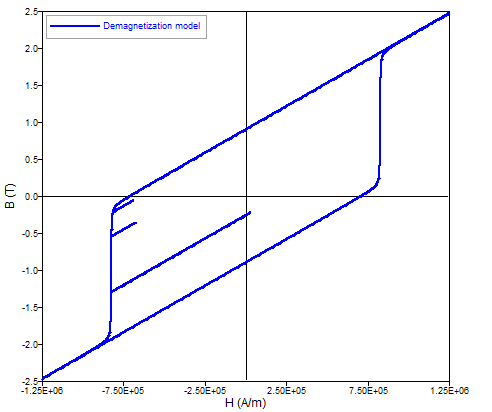# Magnet (unidirectional): demagnetization curve (Hc, Br)

## Presentation

This model ( Nonlinear magnet described by Hc and the Br module ) defines a nonlinear B(H) dependence with taking into account of demagnetization, wherever the curve knee is.

Main characteristics:

• the mathematical model and the direction of magnetization are dissociated
• a single material for description of several regions

## Mathematical model

In the direction of magnetization the model is a combination of a straight line and an arc tangent curve.

The corresponding mathematical formula is written:with:where:

• μ0 is the permeability of vacuum, μ0 = 4 π 10-7 (H/m)
• μrmax is the maximal relative permeability of material
• Br is the remanent flux density (T)
• Js is the saturation magnetization (T)

The shape of the B(H) dependence in the direction of magnetization is given in the opposite figure.In transversal directions one can write:

B(H)= μ0μr⊥H

where μr⊥ is the transverse relative permeability

## Direction of magnetization

The various possibilities provided to the user are the same ones as those presented in § Magnet (unidirectional): linear approximation.

## Demagnetization during solving

With this non-linear model, it is now possible to taking in account demagnetization during solving by checking the thick that is provided for. This model is based on a static Preisach model, and can be applied in all over the B(H) law of the magnet:

• Available for 2D and 3D in magnetic transient application
• Initialization by static calculation (Application > Transient initialization)
• This model does not take in account temperature variationsTo use this new model with a solved project :

• Destroy results
• Go in Application > Transient initialization and select : Initialization by static calculation
• Create a new material Nonlinear magnet describes by Hc and Br module
• Check the thick Taking in account demagnetization during solving
• Assign the material to regions
• Go to Physics > Face regions (in 2D) or Volume regions (in 3D) > Orient material for face / volume regions
• Run the scenario
• Create a new isovalues, select magnet and add BrDemag in the formulas field

## Example of results

Here is a surface permanent magnets motor 3D For a control angle , we can see that there is a local effect on the remanent flux density, but the repartition depends of the angle $\Psi$ .

This local effect can bring some effect on global values like torque or EMF.
Note:
• This new level of modeling can increase the computation time and the memory (ram & disk)
• Not available in 3D with the potential vector and 2D axisymmetric